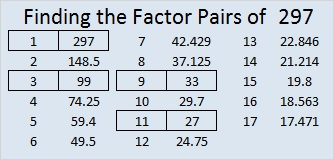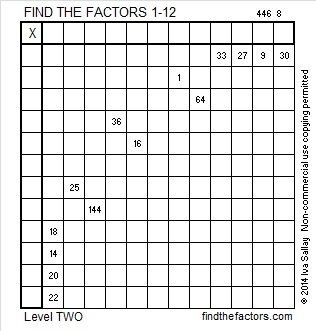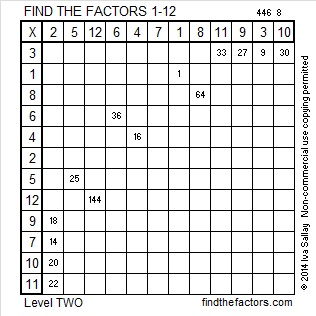# 297 and Level 2

• 297 is a composite number.
• Prime factorization: 297 = 3 x 3 x 3 x 11 which can be written 297 = 3³ x 11
• The exponents in the prime factorization are 3 and 1. Adding one to each and multiplying we get (3 + 1)(1 + 1) = 4 x 2 = 8. Therefore 297 has exactly 8 factors.
• Factors of 297: 1, 3, 9, 11, 27, 33, 99, 297
• Factor pairs: 297 = 1 x 297, 3 x 99, 9 x 33, or 11 x 27
• Taking the factor pair with the largest square number factor, we get √297 = (√9)(√33) = 3√33 ≈ 17.234This multiplication table has only 14 clues. Is that enough to complete it?Print the puzzles or type the factors on this excel file: 12 Factors 2014-11-17## One thought on “297 and Level 2”

1.Steve Morris

Yes it is 🙂

This site uses Akismet to reduce spam. Learn how your comment data is processed.